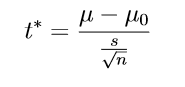# I. A Brief Overview

Consider an example of a courtroom trial:

A car company C is accused of not manufacturing environment-friendly vehicles. The average CO2 emission per car from different manufacturers based on a survey from the previous year is 120.4 grams per kilometer. But for a random batch of 100 cars produced at C’s factory, the average CO2 emission is 121.2 grams per kilometer with a standard deviation of 1.8.

At the trial, Company C is not considered to be guilty as long as their wrongdoing is not proven. A public prosecutor tries to prove that C is guilty and can only succeed when enough evidence is presented.

The example above illustrates the concepts of hypothesis testing; specifically, there are two conflicting hypotheses:

i) C is not guilty; or

ii) C is guilty

The first is called the null hypothesis (denoted by H0), and the second the alternative hypothesis (denoted by HA). At the start of the trial, the null hypothesis is temporarily accepted, until proven otherwise. The goal of hypothesis testing is to perform some sort of transformed comparison between the two numbers 121.2 and 120.4 to either reject H0 and accept HA or vice versa. This one-sample mean testing because we are comparing the average value obtained from one sample (121.2) with the average value assumed to represent the whole population (120.4)

# II. Required Steps for Hypothesis Testing

The six steps below must be followed to conduct a hypothesis test. The details will be elaborated on with our example afterward.

1) Set up null and alternative hypotheses and check conditions.

2) Determine the significance level, alpha.

3) Calculate the test statistic.

4) Calculate the probability value (a.k.a the p-value), or find the rejection region. For the following example, we will use the p-value.

5) Decide on the null hypothesis.

6) State the overall conclusion.

# III. A step-by-step example

## 1) Set up hypotheses:

We already mentioned in the beginning the two hypotheses. But now we will formalize them:

### Null hypothesis:

Company C’s CO2 mean (denoted by μ ) is equal to the population mean (denoted by μ0):           μ = μ0

### Alternative hypothesis:

Company C’s CO2 mean is greater than the population mean: μ > μ0

The hypothesis testing for the one-sample means we are conducting requires the data to come from an approximately normal distribution or a large enough sample size, which can be quite subjective. To keep things simple, we decide that the data gathered from company C is big enough with the sample size being 100 cars.

## 2) Determine the significance level, alpha, or confidence level

The significance level and its complementary, the confidence level, provide a level of probability cutoff for our test to make decisions about the hypotheses. A common value for alpha is 5%, which is the same as a confidence level of 95%.

## 3) Calculate the test statistic

For the one-sample mean test, we calculate the t* test statistic using the formula:where s is the standard deviation from the sample we are testing, 1.8, and n is the size of the sample, 100.